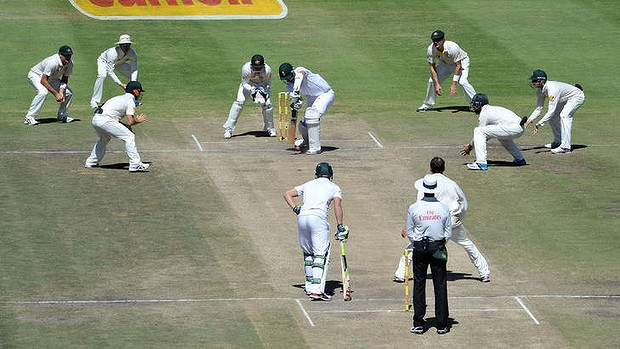# I don't think its a logic problem

Logic Level 3Assuming no extras (wide balls and no balls) and overthrows, what is the maximum number of runs that a batsman can score in two overs if he starts playing from the first ball of the first over?

Details and assumptions :-

$\Rightarrow$ This question assumes that you are familiar with the rules of cricket.

×